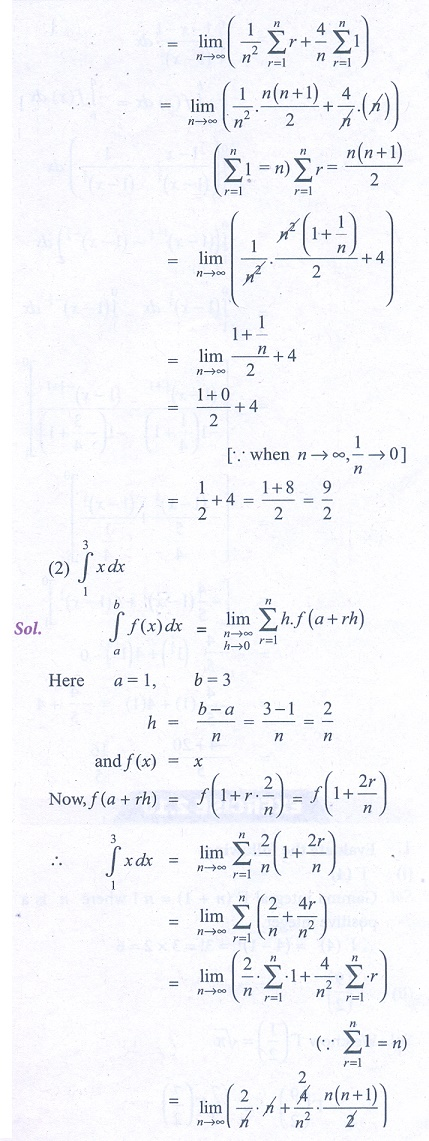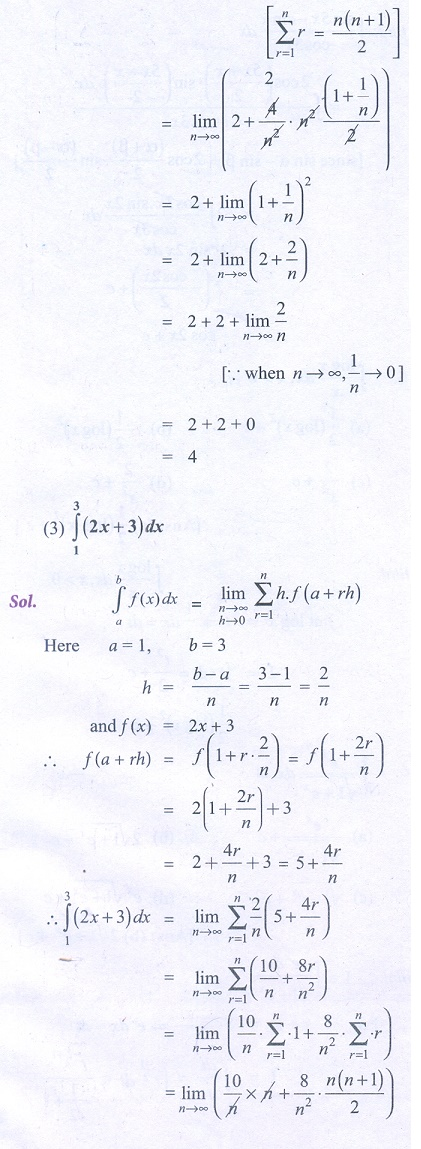Home | | Business Maths 12th Std | Definite integral as the limit of a sum

# Definite integral as the limit of a sum

The following results are very useful in evaluating definite integral as the limit of a sum

Definite integral as the limit of a sum

Let f (x) be a continuous real valued function in [a ,b], which is divided into n equal parts of width h , thenThe following results are very useful in evaluating definite integral as the limit of a sumExampleExercise 2.11

Evaluate the following integrals as the limit of the sum:Tags : Exercise and Example Solved Problems with Answer, Solution , 12th Business Maths and Statistics : Chapter 2 : Integral Calculus - I
Study Material, Lecturing Notes, Assignment, Reference, Wiki description explanation, brief detail
12th Business Maths and Statistics : Chapter 2 : Integral Calculus - I : Definite integral as the limit of a sum | Exercise and Example Solved Problems with Answer, Solution